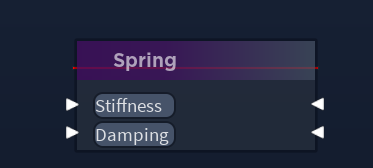# Drawing 2D line between two points

im trying to draw line between two points

the problem is that the line ends up little higher than neededThe red line should be between the two white triangles

``````local module = {}

local gui = script.Parent

local Atan2 = math.atan2
local Pi = math.pi
local Abs = math.abs
local Sqrt = math.sqrt
local Deg = math.deg

local VECTOR2_HALF = Vector2.new(0.5, 0.5)

local function drawLineFromTwoPoints(pointA, pointB)
-- These found from: https://devforum.roblox.com/t/drawing-a-line-between-two-points-in-2d/717808/2
-- Size: sqrt((x1-x2)^2+(y1-y2)^2)
-- Pos: ((x1+x2)/2,(y1+y2)/2)

local lineFrame = Instance.new("Frame")
lineFrame.BackgroundColor3 = Color3.new(1, 0, 0)

lineFrame.Size = UDim2.fromOffset(
Sqrt((pointA.X - pointB.X) ^ 2 + (pointA.Y - pointB.Y) ^ 2),
2
)
lineFrame.Position = UDim2.fromOffset(
(pointA.X + pointB.X) / 2,
(pointA.Y + pointB.Y) / 2
)
lineFrame.Rotation = Deg(Atan2(
(pointA.Y - pointB.Y), (pointA.X - pointB.X)
))

lineFrame.AnchorPoint = VECTOR2_HALF
lineFrame.BorderSizePixel = 1
lineFrame.Parent = script.Parent.Body.MVVM.Nodes
end

function module.drawLineUI(obj1, obj2)
-- Get center regardless of anchor point
local posA = obj1.AbsolutePosition + (obj1.AbsoluteSize * VECTOR2_HALF)
local posB = obj2.AbsolutePosition + (obj2.AbsoluteSize * VECTOR2_HALF)

return drawLineFromTwoPoints(posA, posB)
end

return module
``````

What is PointA and PointB? Also I think your problem is that you are doing it in offset. Doing it in scale should work.

Edit: Try doing this:

``````lineFrame.Position = UDim2.new(
0,
(pointA.X + pointB.X) / 2,
.5,
(pointA.Y + pointB.Y) / 2
)``````

The offset appears to be 36 pixels, which happens to be the CoreGui inset amount. I would check the `.IgnoreGuiInset` property of the ScreenGuis

yes you are right,i had ignoreguiinset on now it works fine